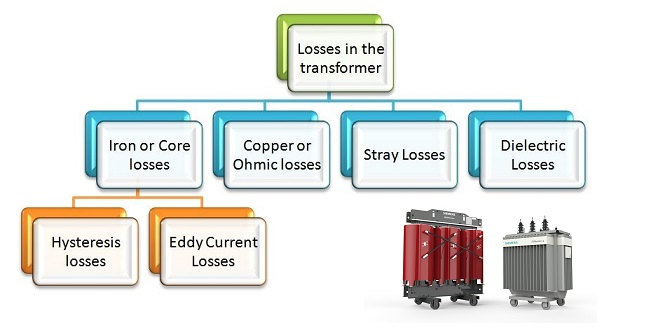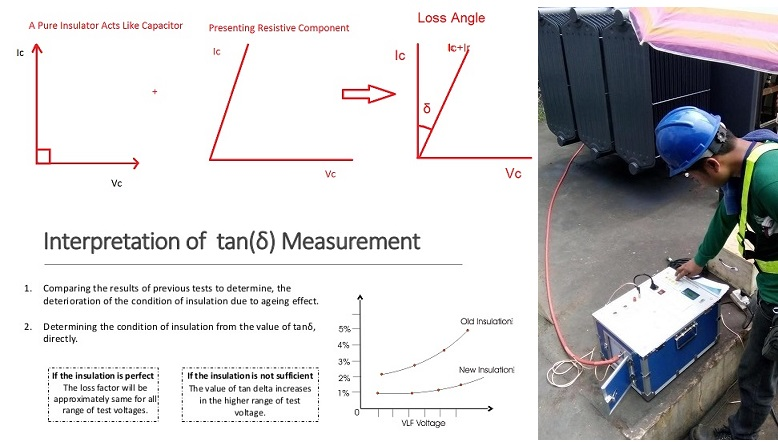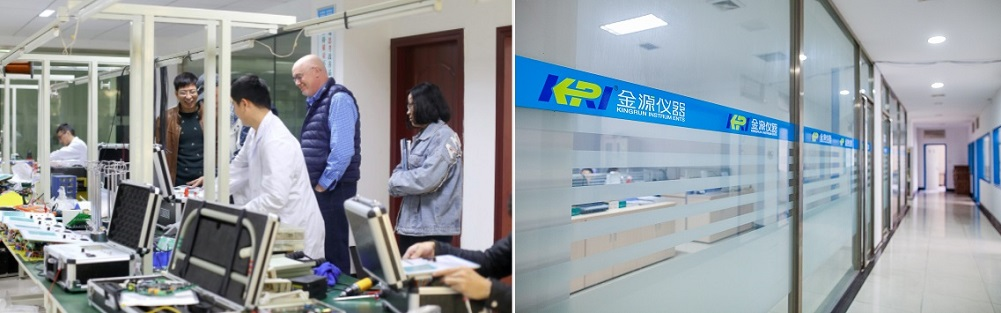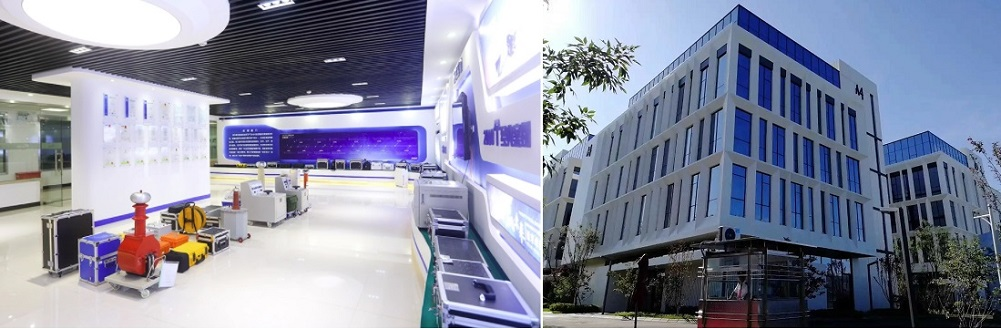Technology Literature

# What is the dielectric loss (Tan Delta) of a transformer and how is it tested?

Dielectric loss: Under the action of an electric field, the energy loss inside the insulating material due to the hysteresis effect of dielectric conductance and dielectric polarization. Also called dielectric loss, referred to as dielectric loss. Under the action of the alternating electric field, the complementary angle δ of the included angle (power factor angle Φ) between the current phaser and the voltage phaser flowing in the dielectric is called the dielectric loss angle.Under the action of the alternating electric field, the charge accumulated in the dielectric has two components:
(1) Active power. One is the power consumed for heating, also known as the in-phase component;

(2) Reactive power, also known as out-of-phase component. The ratio of the out-of-phase component to the in-phase component is called the dielectric loss tangent tanδ. tanδ=1/WCR (where W is the angular frequency of the alternating electric field; C is the dielectric capacitance; R is the loss resistance). The dielectric loss tangent is a dimensionless physical quantity.1. The dielectric loss of the transformer generally refers to the magnetic dielectric loss. It mainly includes two parts: one part is hysteresis loss, and the other part is eddy current loss.

Hysteresis loss

The hysteresis loss is due to the existence of a "hysteresis loop" in the iron core, so that the phase difference between the induced electromotive force and the magnetizing current is not equal to 90 degrees. We know that if it is 90 degrees, the current is "reactive". Now it is not equal to 90 degrees, which is equivalent to connecting an active current component in parallel.

By Steinmetz formula hysteresis loss, Wh =Kh f Bm1.6 w/m3
Kh – hysteresis constant
Eddy Current Loss
It is the result of induced current in the core due to the electromagnetic induction in ferromagnetic material, which flows as loops of electrical current within the conductor.
Eddy Current loss, We = Ke f 2 Bm2 t 2 w/m3
Ke – co-efficient of eddy current
t – Thickness of the sheet

Eddy current loss can be reduced by using an iron core made of thin laminated sheets and material with high electrical resistivity. It reduces the induced EMF and the amount of current flow.

2. Specific explanation:

When the transformer is working normally, the magnetic field generated by the secondary side current and the main part of the primary current are offset. The magnetic field left after cancellation should be roughly equal to the magnetic field at no load (when the secondary current is zero) (assuming resistance and leakage flux are ignored). Therefore, the "magnetizing current" I mentioned above should be equal to the no-load current of the transformer.
Ideally, the no-load current should be 90 degrees behind the voltage, which is "reactive". But with the above losses, this lag is not 90 degrees enough. The complementary angle δ of this lag angle can also represent the size of the loss. The mathematical relationship is very similar to dielectric loss.

When the transformer is no-load, if there is no loss, the input characteristics of the primary side should be equivalent to a pure inductance. With loss, the input current is equivalent to adding a resistor in parallel to the inductor. tanδ should be equal to the ratio of the current on this equivalent resistor to the current on the inductor.Other transformers types:

1. Iron Losses or Core Loss
Iron loss occurs in the transformer core due to the alternating magnetic flux. It consists of eddy current loss and hysteresis loss.

Iron loss = Hysteresis loss + Eddy Current loss

Referring the below equations of hysteresis and eddy current loss,

Both the Eddy current loss and hysteresis loss depend upon the magnetic properties of the core material, f-frequency of the AC supply and the Bm– maximum flux density.
2. Copper Loss
Copper loss occurs due to the ohmic resistance in both primary and secondary winding.
Calculating the copper loss in the primary and secondary winding,

Total Copper Loss, Pcu = I12R1 + I22R2 ;

The values of Resistance R1 of the primary winding and R2 of the secondary winding are constant.

I1 – primary current, I2 – secondary current

Then it is clear from the above equation that the copper loss varies with the amount of current (square of the current) through the windings.
3. Dielectric Loss

It occurs in the insulating material and oil of the transformer. The transformer oils are subject to change its parameters such as dielectric strength, tan delta, moisture, chemical parameters (dissolved impurities – dissolution of copper), physical parameters, etc…

1.Iron loss (Hysteresis loss, eddy current loss) and dielectric loss are no-load losses which are independent of the transformer load.
2.Whereas the copper loss and stray loss varies with the load current.
3.Iron loss is a constant loss and copper loss is a variable loss.
4.Copper loss is determined by the short circuit test and Iron loss is determined by the open circuit test.

The test items are required for 110kV/220kV substation acceptance and maintenance testing(with corresponding tester types):

 Test Object Testing Item Tester Type Cable Cable AC Hipot Testing JYCX Cable Insulation Resistance Testing JYM KYORITSU Transformer Insulation Resistance of Winding JYM KYORITSU DC Leakage Testing in 1min JYDHV Winding Resistance Testing JYR50S Winding Deformation Testing JYP Turns Ratio Testing JYT-A AC Hipot Testing JYCX Short Circuit Impedance Testing JYW6300 Dan Delta Testing JYC Oil Breakdown Voltage (BDV)Testing JY6611 Oil Tan Delta Testing GTD-61A Switchgear Circuit Breaker/Disconnector Resistance JYL-200B Relay Relay Performance JY7003G On-load On-load Switchgear Testing JYK-I Tap Changer Dynamic Testing JYK-I Instrument   Transformer Current Transformer (CT)Testing JYH-C Potential Transformer (PT)Testing JYH-C Surge Arrestor Zinc Oxide Characteristics Testing JY6800 1mA DC Reference Voltage JYDHV Capacitor Capacitor Current JY6700 Capacitor Inductance JY6700 Ground Grid Grounding Down Lead Earth JYD

Related Articles:

DC winding resistance tester with 500 times tests on a single charge -JYR9310